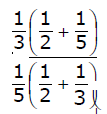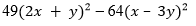# ICSE Class 7 Maths Important Questions

The subject experts at BYJU’S prepare ICSE Class 7 Maths important questions from the latest curriculum or syllabus of Class 7 Maths. Important questions of Class 7 Maths equip students with brief knowledge about the question pattern, essential topics, marking scheme, etc. By following ICSE Class 7 Maths important questions, students can evaluate their performance and preparations. It also helps them in augmenting self-confidence. All the essential concepts and topics of Class 7 Maths are covered while framing the important questions to help the students of ICSE Class 7 to improve their conceptual learning. These questions can also be used as ICSE Class 7 Maths Worksheets.

Going through these important questions will help students understand the type of questions asked in the exam. This will give good practice to students, and they will be able to face the board exam with full preparation. Along with the other study materials, students should also give importance to these important questions of Maths as it is prepared according to the ICSE Class 7 Maths syllabus.

All the ICSE Class 7 Important Questions of Maths are mentioned below so that students can practice them so that they become perfect at solving mathematical problems. Students can practice the pdf question as ICSE Class 7 Maths Worksheets. It will boost their exam preparation and help them to score high marks.

1. If the marks of Sachin, Kohli and Dhoni are in the ratio of 4: 5: 6, and Ajay got 75 marks, then find the marks of Sachin and Dhoni.
2. A train 270 m long is running at 40.5 km/hr. How much time will it take to cross the tree?
3. The given data shows the marks obtained by 20 students of a class in a Math test.
31, 9, 8, 20, 8, 7, 30, 31, 24, 20, 13, 13, 28, 26, 19, 27, 13, 12, 25, 21
Represent the data in a frequency distribution table and find the mean.
4. Ankit’s monthly expenditure is Rs. 15000. He spends 25% on house rent, 40% on food and groceries, 5% each on travelling and entertainment and the rest on education. Calculate the amount he spends on each.
5. The population of a town increases by 6% annually. If the present population is 17490, what was it a year ago?
6. Write the following sets in the roster method.
1. M is a set of first 5 multiples of 3.
2. V is a set of vowels in ‘DISJOINT’.
7. Solve:
1. (−48) × 24 × (−10) + 100
2. (−56) + 27 – 45 – 17 + 19
8. What is the square root of i) 1.44 and ii) 289
9. Solve the inequation: −14 – 5x ≥ 3x + 2, x ∈ integers
10. The ratio of the ages of the two brothers is 3: 2. If the elder brother’s age is 21 years, how old is the younger brother? Find the ratio of their ages after 7 years.
11. Draw a Venn-Diagram to represent A = {2, 4, 6, 8, 10} and B = {4, 8, 12, 16, 20}. Find A ∪ B and A ∩ B.
12. Convert as instructed:
1. 36 km/hr to m/sec
2. 75 cm/sec to m/ sec
13. By selling a dress for Rs. 729, a shopkeeper experienced a loss of 10%. Find the cost price of the dress.
14. Simplify:15. Factorise:16. In a poultry farm, 300 eggs are produced every day. If 100 birds produce an average of 75 eggs each day, how many birds are there in the poultry farm? Also, find how many more birds should be bought if 375 eggs are needed every day.
17. The H.C.F. of two numbers is 18, and their L.C.M. is 108. One of the numbers is 54. Find the other number.
18. Multiply: 53 × 67
19. Each interior angle of a polygon is 140°. Find the number of sides.
20. Aryan has a rectangular garden whose length is double its width. The area of the garden is 450 square cm. What is the length of the garden?

### Benefits of practising ICSE Class 7 Maths Important Questions

• It helps students to go through all the crucial topics.
• ICSE Important Questions helps in doing a quick revision of the entire syllabus just before the final exam.
• It provides all the important numerical problems and formulas from each chapter.
• It boosts students’ overall confidence levels.

Did you find “ICSE Class 7 Maths Important Questions” useful for your studies? Do let us know your view in the comment section. Keep Learning, and don’t forget to download the BYJU’S App for more interesting study videos.